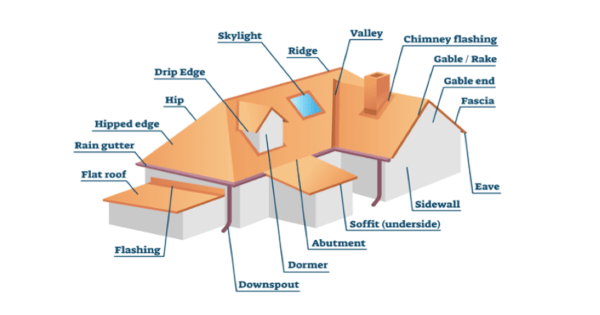## How to Calculate Roof Area – DIY

Multiply your house length by your house width to get the area. (For example, 40 feet x 30 feet = 1,200 square feet.) Next, multiply the area by your roof’s pitch. (1,200 x 1.05 = 1,260 square feet.)

### Calculate the exact roof area

Follow these steps to figure out the exact area of your roof:

• Take your pitch number and divide it by 12. For example, if your pitch is 4 in 12, you need to divide 4 by 12. The answer will be 1/3.
• Next, you need to square your outcome. In our example, 1/3 squared will yield 1/9.
• Calculate the square root of your new number: The square root of 10/9 is 1.05.
• Measure the length of your house – including the overhangs.
• Measure the width of your house – including the overhangs.
• Multiply the length of your house by its width to get the area. For example, 40 feet x 20 feet = 1,200 square feet.
• Thereafter, multiply the area by the pitch to get the true roof area: 1,200 x 1.05 = 1,260 square feet.

In order to allow for ridges, hips, and waste, it is advisable to add 10% of your final roof area for a gable roof or 17% in the case of a cottage roof. Follow Us!

As a result, you should be working with 1,386 sq. feet or 1,474 sq. feet for a gable roof or a cottage roof respectively. Get to know more about us!There are three main types of roofs common on most of the homes in the Dallas area. There is the one-plained square or rectangle shed roofs that can be measured up in seconds. Another common roof is the gable which goes up one side, meets in a triangle, and then slopes down the back side. These are also easy to measure but involve a little bit of adding for the total square foot. Hip roofs are the third type and are a little trickier because they feature four triangle-typed shapes that angle up towards a central peak.

##### Get a Friend, Get On the Roof, and Measure Your Roof

The first way to measure your own roof involves physically climbing up with your tape and taking down the measurements. Roofs are measured in square foot so if you had a simple rectangle shed that was 12′ x 12′ you’d have 144 square feet you need shingled. Finding the square footage of a gable roof works in much of the same way, if the two plains are both 15′ X 30′ you’d have 450 square feet on both sides, or 900 square feet total.

Hip roofs require some geometric calculating to get the proper square footage. Generally hip roofs feature two trapezoids and two triangles. To calculate the area of a trapezoid you add the length of the bottom base plus the length of the top base and multiply it by the height. You take that number and divide it by 2 to get the area of one trapezoid base. The area of a triangle is calculated by taking the base multiplied by the height and dividing by two. Hip roof square footage is then figured by adding all the sides together.## Roofing Materials

The purpose of calculating the precise measurements of a roof is to ensure that the appropriate amount of roofing materials are ordered – whether you have a shingles roof, gable roof or metal roof. Here are some common roofing materials and considerations for each:

#### Shingles

Shingles are typically sold in bundles. Depending on the type and brand of shingle, it may take a different number of bundles to make a square. When cost comparing shingles, remember to base price on squares and not on bundles.

#### Metal Roofing

Metal roofing is typically sold by the sheet or panel. To determine the number of sheets or panels necessary to cover the roof, divide the width of the roof by the sheet or panel coverage width.

#### Other Roofing Materials

The basic square footage and length calculations detailed above should help estimate overall cost for other materials. Additional roofing materials that should be accounted for include items such as leak barrier, deck protection, drip edge, rake edge, ridge vents, and hip and ridge caps.

0